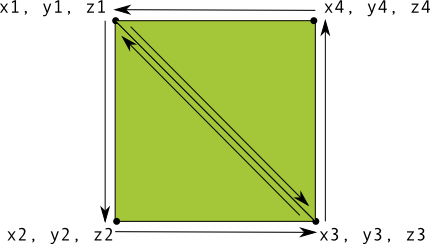# Define shapes

Being able to define shapes to be drawn in the context of an OpenGL ES view is the first step in creating high-end graphics for your app. Drawing with OpenGL ES can be a little tricky without knowing a few basic things about how OpenGL ES expects you to define graphic objects.

This lesson explains the OpenGL ES coordinate system relative to an Android device screen, the basics of defining a shape, shape faces, as well as defining a triangle and a square.

## Define a triangle

OpenGL ES allows you to define drawn objects using coordinates in three-dimensional space. So, before you can draw a triangle, you must define its coordinates. In OpenGL, the typical way to do this is to define a vertex array of floating point numbers for the coordinates. For maximum efficiency, you write these coordinates into a `ByteBuffer`, that is passed into the OpenGL ES graphics pipeline for processing.

### Kotlin

```// number of coordinates per vertex in this array
const val COORDS_PER_VERTEX = 3
var triangleCoords = floatArrayOf(     // in counterclockwise order:
0.0f, 0.622008459f, 0.0f,      // top
-0.5f, -0.311004243f, 0.0f,    // bottom left
0.5f, -0.311004243f, 0.0f      // bottom right
)

class Triangle {

// Set color with red, green, blue and alpha (opacity) values
val color = floatArrayOf(0.63671875f, 0.76953125f, 0.22265625f, 1.0f)

private var vertexBuffer: FloatBuffer =
// (number of coordinate values * 4 bytes per float)
ByteBuffer.allocateDirect(triangleCoords.size * 4).run {
// use the device hardware's native byte order
order(ByteOrder.nativeOrder())

// create a floating point buffer from the ByteBuffer
asFloatBuffer().apply {
// add the coordinates to the FloatBuffer
put(triangleCoords)
// set the buffer to read the first coordinate
position(0)
}
}
}
```

### Java

```public class Triangle {

private FloatBuffer vertexBuffer;

// number of coordinates per vertex in this array
static final int COORDS_PER_VERTEX = 3;
static float triangleCoords[] = {   // in counterclockwise order:
0.0f,  0.622008459f, 0.0f, // top
-0.5f, -0.311004243f, 0.0f, // bottom left
0.5f, -0.311004243f, 0.0f  // bottom right
};

// Set color with red, green, blue and alpha (opacity) values
float color[] = { 0.63671875f, 0.76953125f, 0.22265625f, 1.0f };

public Triangle() {
// initialize vertex byte buffer for shape coordinates
ByteBuffer bb = ByteBuffer.allocateDirect(
// (number of coordinate values * 4 bytes per float)
triangleCoords.length * 4);
// use the device hardware's native byte order
bb.order(ByteOrder.nativeOrder());

// create a floating point buffer from the ByteBuffer
vertexBuffer = bb.asFloatBuffer();
// add the coordinates to the FloatBuffer
vertexBuffer.put(triangleCoords);
// set the buffer to read the first coordinate
vertexBuffer.position(0);
}
}
```

By default, OpenGL ES assumes a coordinate system where [0,0,0] (X,Y,Z) specifies the center of the `GLSurfaceView` frame, [1,1,0] is the top right corner of the frame and [-1,-1,0] is bottom left corner of the frame. For an illustration of this coordinate system, see the OpenGL ES developer guide.

Note that the coordinates of this shape are defined in a counterclockwise order. The drawing order is important because it defines which side is the front face of the shape, which you typically want to have drawn, and the back face, which you can choose to not draw using the OpenGL ES cull face feature. For more information about faces and culling, see the OpenGL ES developer guide.

## Define a square

Defining triangles is pretty easy in OpenGL, but what if you want to get a just a little more complex? Say, a square? There are a number of ways to do this, but a typical path to drawing such a shape in OpenGL ES is to use two triangles drawn together:Figure 1. Drawing a square using two triangles.

Again, you should define the vertices in a counterclockwise order for both triangles that represent this shape, and put the values in a `ByteBuffer`. In order to avoid defining the two coordinates shared by each triangle twice, use a drawing list to tell the OpenGL ES graphics pipeline how to draw these vertices. Here’s the code for this shape:

### Kotlin

```// number of coordinates per vertex in this array
const val COORDS_PER_VERTEX = 3
var squareCoords = floatArrayOf(
-0.5f,  0.5f, 0.0f,      // top left
-0.5f, -0.5f, 0.0f,      // bottom left
0.5f, -0.5f, 0.0f,      // bottom right
0.5f,  0.5f, 0.0f       // top right
)

class Square2 {

private val drawOrder = shortArrayOf(0, 1, 2, 0, 2, 3) // order to draw vertices

// initialize vertex byte buffer for shape coordinates
private val vertexBuffer: FloatBuffer =
// (# of coordinate values * 4 bytes per float)
ByteBuffer.allocateDirect(squareCoords.size * 4).run {
order(ByteOrder.nativeOrder())
asFloatBuffer().apply {
put(squareCoords)
position(0)
}
}

// initialize byte buffer for the draw list
private val drawListBuffer: ShortBuffer =
// (# of coordinate values * 2 bytes per short)
ByteBuffer.allocateDirect(drawOrder.size * 2).run {
order(ByteOrder.nativeOrder())
asShortBuffer().apply {
put(drawOrder)
position(0)
}
}
}
```

### Java

```public class Square {

private FloatBuffer vertexBuffer;
private ShortBuffer drawListBuffer;

// number of coordinates per vertex in this array
static final int COORDS_PER_VERTEX = 3;
static float squareCoords[] = {
-0.5f,  0.5f, 0.0f,   // top left
-0.5f, -0.5f, 0.0f,   // bottom left
0.5f, -0.5f, 0.0f,   // bottom right
0.5f,  0.5f, 0.0f }; // top right

private short drawOrder[] = { 0, 1, 2, 0, 2, 3 }; // order to draw vertices

public Square() {
// initialize vertex byte buffer for shape coordinates
ByteBuffer bb = ByteBuffer.allocateDirect(
// (# of coordinate values * 4 bytes per float)
squareCoords.length * 4);
bb.order(ByteOrder.nativeOrder());
vertexBuffer = bb.asFloatBuffer();
vertexBuffer.put(squareCoords);
vertexBuffer.position(0);

// initialize byte buffer for the draw list
ByteBuffer dlb = ByteBuffer.allocateDirect(
// (# of coordinate values * 2 bytes per short)
drawOrder.length * 2);
dlb.order(ByteOrder.nativeOrder());
drawListBuffer = dlb.asShortBuffer();
drawListBuffer.put(drawOrder);
drawListBuffer.position(0);
}
}
```

This example gives you a peek at what it takes to create more complex shapes with OpenGL. In general, you use collections of triangles to draw objects. In the next lesson, you learn how to draw these shapes on screen.

[]
[]